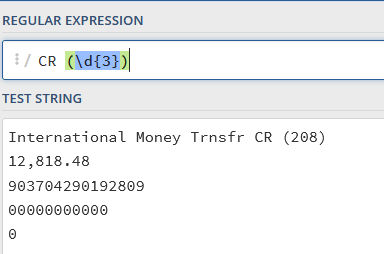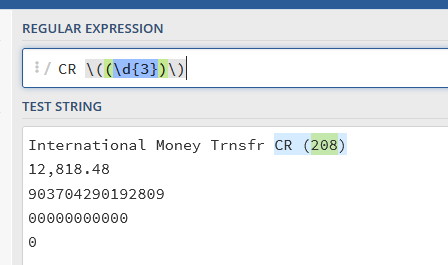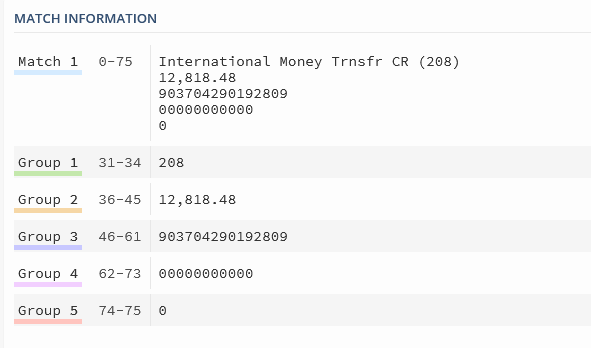# Regular Expreesions

There is a part of data that we are trying to extract from pdf. We are doing it using Regex. Problem we are facing is
we are able to match the Regex with the string online, its giving exact match. But when we are applying the same in studio,
we are not able to get the match.

The text is →

International Money Trnsfr CR (208)
12,818.48
903704290192809
00000000000
0

Regex we are using is:

[a-zA-Z ]+ Money [a-zA-Z ]+ (\d{3})+(\n)+(\d+|\d{1,3}(,\d{3})*)(.\d+)( |\S|\n)+\d{15}( |\n)+\d{1,12}(\n\d)+

The string is different is visibility in pdf…when converted to text its different.
When we see in PDF it looks like

International Money Trnsfr CR (208) 12,818.48 903704290192809 00000000000

But when code runs, and we write the data to text file to check it is in the below format:

International Money Trnsfr CR (208)
12,818.48
903704290192809
00000000000
0

give a try on adding conditional \r?

[a-zA-Z ]+ Money [a-zA-Z ]+ ((\d{3}))+(\r?\n)+(\d+|\d{1,3}(,\d{3})*)(.\d+)( |\S|\r?\n)+\d{15}( |\r?\n)+\d{1,12}(\r?\n\d)+

but also check as (\d{3}) will not match (208) due surrounding Bracket are definedvsAs alternate:Duplicate of the below post…

1 Like